# Capacitance

Next Page: Impedance and Reactance

Also See: Capacitors | Power Supplies

## Capacitance

Capacitance (symbol C) is a measure of a capacitor's ability to store charge. A large capacitance means that more charge can be stored. Capacitance is measured in farads, symbol F, but 1F is very large so prefixes (multipliers) are used to show smaller values:

• µ (micro) means 10-6 (millionth), so 1000000µF = 1F
• n (nano) means 10-9 (thousand-millionth), so 1000nF = 1µF
• p (pico) means 10-12 (million-millionth), so 1000pF = 1nF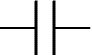unpolarised capacitor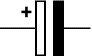polarised capacitor

Rapid Electronics: Capacitors

## Charge and Energy Stored

The amount of charge (Q) stored by a capacitor is given by:

 Charge,   Q = C × V

When they store charge, capacitors are also storing energy (E):

 Energy,   E = ½QV = ½CV²

Q = charge in coulombs (C)
C = capacitance in farads (F)
V = voltage in volts (V)
E = energy in joules (J)

#### Capacitors return their stored energy to the circuit

Note that capacitors return their stored energy to the circuit. They do not 'use up' electrical energy by converting it to heat as a resistor does.

The energy stored by a capacitor is much smaller than the energy stored by a battery so they cannot be used as a source of energy for most purposes.

## Capacitive Reactance Xc

Capacitive reactance (Xc) is a measure of a capacitor's opposition to AC (alternating current). Like resistance it is measured in ohms (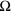) but reactance is more complex than resistance because its value depends on the frequency (f) of the electrical signal passing through the capacitor as well as on the capacitance (C).

 Capacitive reactance,   Xc = 1 2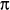fC

Xc = reactance in ohms ()
f    = frequency in hertz (Hz)
C  = capacitance in farads (F)

The reactance is large at low frequencies and small at high frequencies. For steady DC which is zero frequency, Xc is infinite (total opposition), hence the rule that capacitors pass AC but block DC.

For example a 1µF capacitor has a reactance of 3.2kfor a 50Hz signal, but when the frequency is higher at 10kHz its reactance is only 16.

#### Capacitive and Inductive Reactance

The symbol Xc is used to distinguish capacitive reactance from inductive reactance XL which is a property of inductors.

The distinction is important because XL increases with frequency (the opposite of Xc) and if both XL and Xc are present in a circuit the combined reactance (X) is the difference between them.

For further information please see the page on Impedance.

## Capacitors in Series and Parallel

The combined capacitance (C) of capacitors connected in series is given by:

 1 = 1 + 1 + 1 + ... C C1 C2 C3

The combined capacitance (C) of capacitors connected in parallel is:

 C = C1 + C2 + C3 + ...

Two or more capacitors are rarely deliberately connected in series in real circuits, but it can be useful to connect capacitors in parallel to obtain a very large capacitance, for example to smooth a power supply.

Note that these equations are the opposite way round for resistors in series and parallel.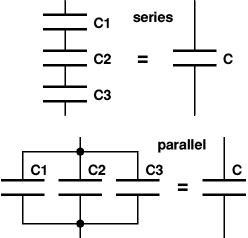## Charging a capacitor

The capacitor (C) in the circuit diagram is being charged from a supply voltage (Vs) with the current passing through a resistor (R). The voltage across the capacitor (Vc) is initially zero but it increases as the capacitor charges. The capacitor is fully charged when Vc = Vs.

The charging current (I) is determined by the voltage across the resistor (Vs - Vc):

 Charging current,  I = (Vs - Vc) / R

At first Vc = 0V so:

 Initial current, Io = Vs / R

Vc increases as soon as charge (Q) starts to build up (Vc = Q/C), this reduces the voltage across the resistor and therefore reduces the charging current. This means that the rate of charging becomes progressively slower.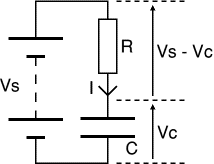## Time constant (RC)

The time constant is a measure of how slowly a capacitor charges with current flowing through a resistor. A large time constant means the capacitor charges slowly. Note that the time constant is a property of the circuit containing the capacitor and resistor, it is not a property of the capacitor alone.

 time constant  = R × C

time constant is in seconds (s)
R = resistance in ohms ()
C = capacitance in farads (F)

#### Examples

If R = 47k& C = 22µF, then RC = 47k× 22µF = 1.0s.
If R = 33k& C = 1µF, then RC = 33k× 1µF = 33ms.

The time constant (RC) is the time taken for the charging (or discharging) current (I) to fall to 1/e of its initial value (Io). 'e' is an important number in mathematics (like). e = 2.71828 (to 6 significant figures) so we can roughly say that the time constant is the time taken for the current to fall to 1/3 of its initial value.

After each time constant the current falls by 1/e (about 1/3). After 5 time constants (5RC) the current has fallen to less than 1% of its initial value and we can reasonably say that the capacitor is fully charged, but in fact the capacitor takes for ever to charge fully!

The bottom graph shows how the voltage (V) increases as the capacitor charges. At first the voltage changes rapidly because the current is large; but as the current decreases, the charge builds up more slowly and the voltage increases more slowly.

 Time Voltage Charge 0RC 0.0V 0% 1RC 5.7V 63% 2RC 7.8V 86% 3RC 8.6V 95% 4RC 8.8V 98% 5RC 8.9V 99%

Charging a capacitor
time constant = RC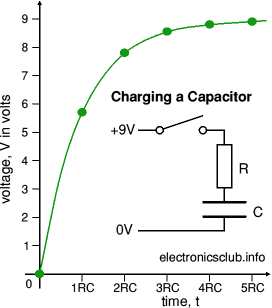After 5 time constants (5RC) the capacitor is almost fully charged with its voltage almost equal to the supply voltage. We can reasonably say that the capacitor is fully charged after 5RC, although really charging continues for ever (or until the circuit is changed).

## Discharging a capacitor

The top graph shows how the current (I) decreases as the capacitor discharges. The initial current (Io) is determined by the initial voltage across the capacitor (Vo) and resistance (R):

 Initial current, Io = Vs / R

Note that the current graphs are the same shape for both charging and discharging a capacitor. This type of graph is an example of exponential decay.

The bottom graph shows how the voltage (V) decreases as the capacitor discharges.

 Time Voltage Charge 0RC 9.0V 100% 1RC 3.3V 37% 2RC 1.2V 14% 3RC 0.4V 5% 4RC 0.2V 2% 5RC 0.1V 1%

Discharging a capacitor
time constant = RC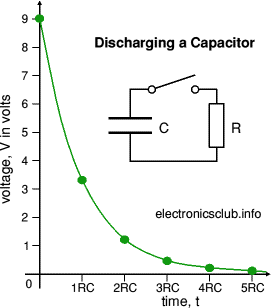At first the current is large because the voltage is large, so charge is lost quickly and the voltage decreases rapidly. As charge is lost the voltage is reduced making the current smaller so the rate of discharging becomes progressively slower.

After 5 time constants (5RC) the voltage across the capacitor is almost zero and we can reasonably say that the capacitor is fully discharged, although really discharging continues for ever (or until the circuit is changed).

## Uses of Capacitors

Capacitors are used for several purposes:

## Capacitor Coupling (CR-coupling)

Sections of electronic circuits may be linked with a capacitor because capacitors pass AC (changing) signals but block DC (steady) signals. This is called capacitor coupling or CR-coupling.

It is used between the stages of an audio system to pass on the audio signal (AC) without any steady voltage (DC) which may be present, for example to connect a loudspeaker. It is also used for the 'AC' switch setting on an oscilloscope.

The precise behaviour of a capacitor coupling is determined by its time constant (RC). Note that the resistance (R) may be inside the next circuit section rather than a separate resistor.

For successful capacitor coupling in an audio system the signals must pass through with little or no distortion. This is achieved if the time constant (RC) is larger than the time period (T) of the lowest frequency audio signals required (typically 20Hz, T = 50ms).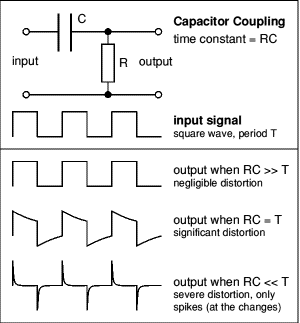• Output when RC >> T
When the time constant is much larger than the time period of the input signal the capacitor does not have sufficient time to significantly charge or discharge, so the signal passes through with negligible distortion.
• Output when RC = T
When the time constant is equal to the time period you can see that the capacitor has time to partly charge and discharge before the signal changes. As a result there is significant distortion of the signal as it passes through the CR-coupling. Notice how the sudden changes of the input signal pass straight through the capacitor to the output.
• Output when RC << T
When the time constant is much smaller than the time period the capacitor has time to fully charge or discharge after each sudden change in the input signal. Effectively only the sudden changes pass through to the output and they appear as 'spikes', alternately positive and negative. This can be useful in a system which must detect when a signal changes suddenly, but ignore slow changes.

Next Page: Impedance and Reactance | Study